## Completion Of Incomplete Pattern

#### Completion Of Incomplete Pattern

Direction: In each of the following questions, find out which of the answer figures (a), (b), (c) and (d) completes the figure?

1. Following question has four alternatives, among which one completes the figures.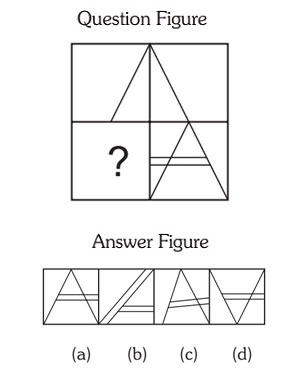1. Right Side figure contains the complete triangle with double across.##### Correct Option: A

Right Side figure contains the complete triangle with double across. So the (a) option in given figure will complete the figures.

1. Select the correct figure from the given Answer Figure that would complete the Question figure.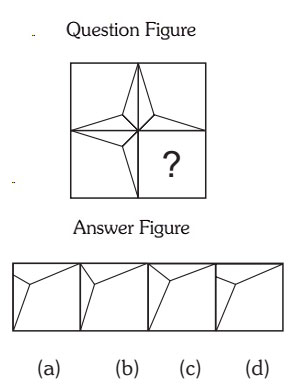1. .

##### Correct Option: C

Figure (c) will complete the figure if you check carefully.

1. Select the correct figure from the Answer Figure that would complete the Question Figure.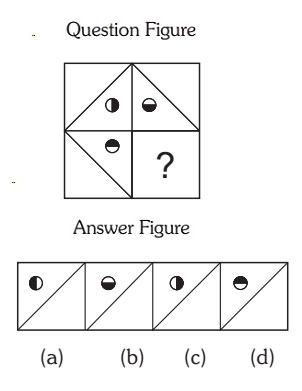1. figure inside the box moves 90 degree in clockwise direction each time.

##### Correct Option: A

figure inside the box moves 90 degree in clockwise direction each time. So if you move the 3rd box figure in clockwise direction with 90 degree, we will get the option (a) is right figure to complete.

1. Following Question has four alternatives, among which one completes the figures.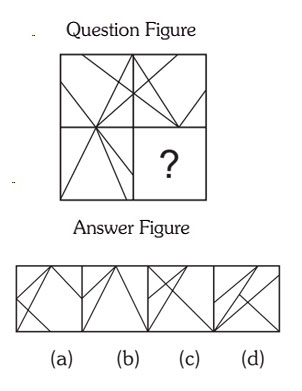##### Correct Option: B

Answer B will complete the figure.

1. Following Question has four alternatives, among which one completes the figures.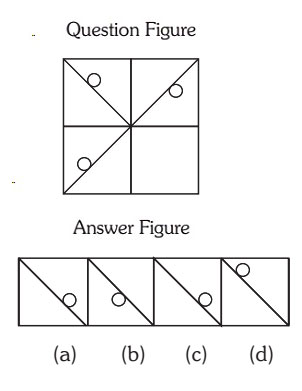1. Rod is moving 90 degree in clockwise direction.

##### Correct Option: B

Rod is moving 90 degree in clockwise direction. Move the Rod from 3rd box by 90 degree in clockwise direction.